# 1. ArchaeoPhases : Introduction

## Introduction

ArchaeoPhases provides a list of functions for the statistical analysis of archaeological dates and groups of dates. It is based on the post-processing of the Markov Chains whose stationary distribution is the posterior distribution of a series of dates. Such MCMC output can be simulated by different applications as for instance 'ChronoModel' (see http://www.chronomodel.fr), 'Oxcal' (see https://c14.arch.ox.ac.uk/oxcal.html) or BCal (see http://bcal.shef.ac.uk/). The only requirement is to have a CSV file containing a sample from the posterior distribution.

## The Graphical User Interface

For those who already know how to use R, ArchaeoPhases won't be difficult to use. For the others, a Graphical User Interface is available. Click here to launch it.

## Installing 'ArchaeoPhases' package

To install ArchaeoPhases you first need to install R and Rstudio that has a nice desktop environment for using R. Once in R (or in RStudio) you can type:

``````install.packages('ArchaeoPhases')
``````

at the R command prompt to install ArchaeoPhases If you then type:

``````library(ArchaeoPhases)
``````

it will load in all the ArchaeoPhases functions.

## Launching the Graphical User Interface from R on localhost

The web shiny application can be launched by the following code :

``````app_ArchaeoPhases()
``````

Here is a live demo.

## Importing data into R

Data files can be imported into R by the following code :

``````data_MCMC = ImportCSV()
``````

In order to use the other functions of the package 'ArchaeoPhases', the date format of the MCMC samples has to be in calendar year.

### Importing data from 'ChronoModel'

Two different files are generated by ChronoModel : “events.csv” that contains the MCMC samples of each event created in the modelling, and “phases.csv” that contains all the MCMC samples of the minimum and the maximum of each group of dates if at least one group is created. Here is an example of the use of the function ImportCSV() for MCMC generated by ChronoModel.

``````ChronoModel_MCMC = ImportCSV("pathToFiles/events.csv", iterationColumn = 1)
``````

The parameter iterationColumn will withdraw the iteration column from the dataframe.

``````ChronoModel_MCMC_Groups = ImportCSV("pathToFiles/phases.csv", iterationColumn = 1)
``````

### Importing data from 'Oxcal'

Oxcal generates a CSV file containing the MCMC samples of all parameters (dates, start and end of phases).

``````Oxcal_MCMC = ImportCSV("pathToFiles/fileName.csv", iterationColumn = 1)
``````

However, the minimum and the maximum of a group of dates can not be extracted from Oxcal. In order to create a dataframe containing these values, use the function CreateMinMaxGroup(). Here is an example of its use:

``````data("KADatesOxcal")
Oxcal_MCMC_Groups = CreateMinMaxGroup(KADatesOxcal, position = 4, name = "IUP")
Oxcal_MCMC_Groups = CreateMinMaxGroup(KADatesOxcal, position = 26, name = "EPI", add=Oxcal_MCMC_Groups, exportFile = "Oxcal_MCMC_Groups.csv")
``````

### Importing data from 'BCal'

BCal generates a CSV file containing the MCMC samples of all parameters (dates, start and end of phases). However, all dates are in format cal BP, that is in year before 1950. Hence, the MCMC have to be converted from the date format cal BP into the calendar year. This can be done by the following lines :

``````BCal_MCMC = ImportCSV("pathToFiles/fileName.csv", iterationColumn = 1, referenceYear = 1950, rowToWithdraw = "last", bin.width=1)
# equivalent to
BCal_MCMC = ImportCSV.BCal("pathToFiles/fileName.csv", bin.width=1)
``````

Note that the last line of the file may contain “NA”. It that case, this line should be withdrawn from the dataset in order to proceed further. Now, again, a file containing the minimum and the maximum values of each group of dates should be created. Let's use the Fishpond dataframe.

``````data("Fishpond")
BCal_MCMC_Groups = CreateMinMaxGroup(Fishpond, position = c(3:6), name = "Layer.II")
BCal_MCMC_Groups = CreateMinMaxGroup(Fishpond, position = 9, name = "Layer.III", add=BCal_MCMC_Groups, exportFile = "BCal_MCMC_Groups.csv")
``````

Note that using the Fichpond dataset, the MCMC samples are in date format cal BP. See example(ImportCSV()) for the convertion of this dataset.

## Convergence of MCMC chains

Let's use the data of Ksar Akil generated by ChronoModel : “KADatesChronoModel” and “KAPhasesChronoModel”. For a more detail on the diagnostic of Markov chain, see Robert and Casella (2009).

To assess the agreement between the posterior distributions and the numerical approximations, three Markov chains were run in parallel by ChronoModel. For each chain, 1 000 iterations were used during the Burn-in period, 20 batches of 500 iterations were used in the Adapt period, 100 000 iterations were drawn in the Acquire period by only 1 out of 10 were kept in order to break the correlation structure.

From the analysis of the history plot, all Markov chains reach their equilibrium before the Acquire period. The autocorrelations of the three Markov chains are not significant, meaning the rate of subsample (1 over 10) is enough.

Now, using the package 'ArchaeoPhases' and the package 'coda', we can verify whether the MCMC samples are correctly generated by the software. Indeed, the MCMC samples should have no autocorrelation and should have reached their equilibrium (that is the posterior density of the parameter under investigation).

``````data("KADatesChronoModel")
mcmcList = coda.mcmc(KADatesChronoModel, numberChains = 3, iterationColumn = 1)
autocorr.plot(mcmcList[,1,])
``````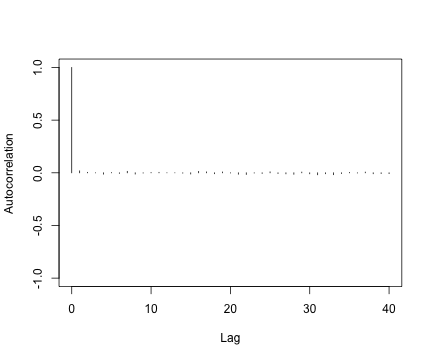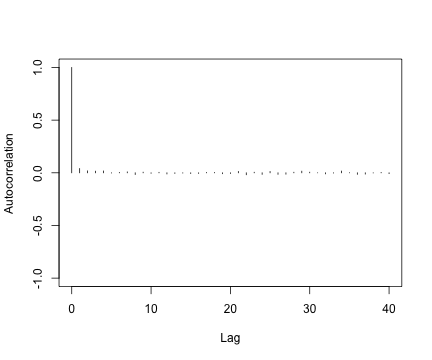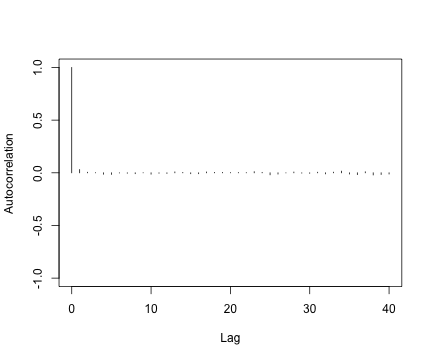The autocorrelation plots show that each of these three chains are not significant. That means that we actually generated a non correlated sample, which was the aim the MCMC process.

We can also check whether the chains reached equilibrium. For example, let's consider the first date of the dataset.

``````plot(mcmcList[,1,])
``````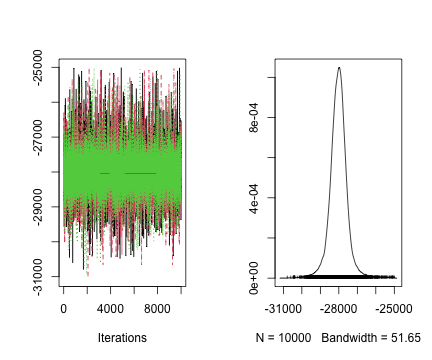The plot shows that the three chains corresponding to the first date reached the same stationnary process.

We can test the Gelman-Rubin criterion. The expected value to confirm that all of the Markov chains reached equilibrium is 1.

``````gelman.diag(mcmcList)
``````
``````Potential scale reduction factors:

Point est. Upper C.I.
Layer.V               1          1
Layer.VI              1          1
[ getOption("max.print") est atteint -- 14 lignes omises ]

Multivariate psrf

1
``````
``````gelman.plot(mcmcList[,1,])
``````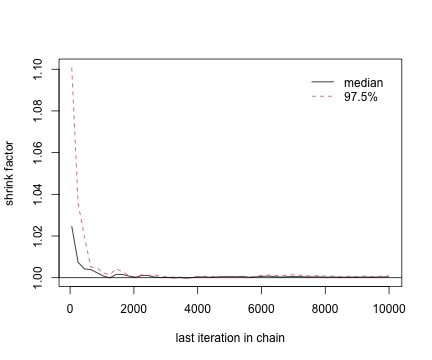The Gelman-Rubin criterion confirms that all of the Markov chains reached equilibrium. We can also test the Geweke criterion. The expected value to confirm that all of the Markov chains reached equilibrium is strickly less than 1.

``````geweke.diag(mcmcList[,1,], frac1=0.1, frac2=0.5)
``````
``````[]

Fraction in 1st window = 0.1
Fraction in 2nd window = 0.5

var1
-0.9288

[]

Fraction in 1st window = 0.1
Fraction in 2nd window = 0.5

var1
0.2023

[]

Fraction in 1st window = 0.1
Fraction in 2nd window = 0.5

var1
-1.583
``````

The Geweke criterion criterion confirms that all of the Markov chains reached equilibrium. As a conclusion, ChronoModel generated correct samples of the posterior distribution. Now gathering the three chains, a total of 30 000 iterations was collected in order to give estimations of the posterior distribution of each parameter.

## References

For a description of the statiscal aspects of the functions implemented in ArchaeoPhases version 1.4 : Anne Philippe, Marie-Anne Vibet. (2020). Analysis of Archaeological Phases using the CRAN Package 'ArchaeoPhases'. . Journal of Statistical Software, Code Snippets, 93, 1–25.

For a use of the tempo plot defined by Dye : Dye, T.S. (2016). Long-term rhythms in the development of Hawaiian social stratification. Journal of Archaeological Science, 71, 1–9

For more details on the diagnostic of Markov chain : Robert and Casella (2009). Introducing Monte Carlo Methods with R. Springer Science & Business Media.

For more details on the Ksar Akil site : Bosch, M. et al. (2015) New chronology for Ksar Akil (Lebanon) supports Levantine route of modern human dispersal into Europe. Proceedings of the National Academy of Sciences, 112, 7683–6.